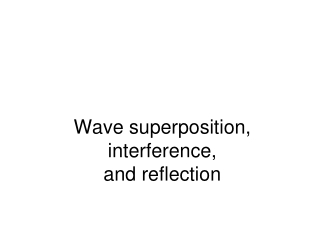Download PresentationWave superposition, interference, and reflection

# Wave superposition, interference, and reflection

Download Presentation## Wave superposition, interference, and reflection

- - - - - - - - - - - - - - - - - - - - - - - - - - - E N D - - - - - - - - - - - - - - - - - - - - - - - - - - -
##### Presentation Transcript

1. Wave superposition, interference, and reflection

2. Adding waves: superposition • When two waves are incident on the same place at the same time, their amplitudes can usually just be added. • In the next few slides we will look at some special cases: (both with two waves at the same frequency and with the same amplitude) • same frequency and amplitude • superposition at a point in space • same direction, but different phase • opposite directions

3. Adding waves • In most simple systems, the amplitude of two waves that cross or overlap can just be added together.

4. Adding two equal amplitude waves at a point

5. For which phase difference  is the superposition amplitude a maximum? • =0 • =/2 • = 

6. For which phase difference  is the superposition amplitude a minimum? • =0 • =/2 • = 

7. Adding two waves that have the same frequency and direction, but different phase • What happens when the phase difference is 0? • What happens when the phase difference is /2? • What happens when the phase difference is ?

8. Constructive interference when =0. • Destructive interference when =. Link to animation of two sine waves adding in and out of phase (Kettering)

9. What happens when a wave is incident on an immovable object? • It reflects, travelling in the opposite direction and upside down. (Now watch patiently while your instructor plays with the demonstration.)

10. Same frequency, opposite directions • What happens when kx is 0? • What happens when kx is /2? • What happens when kx is ? Link to animation of two sine waves traveling in opposite directions

11. Standing waves • Interfering waves traveling in opposite directions can produce fixed points called nodes. • y1= ym sin(kx – wt) • y2= ym sin(kx + wt) v=w/k • yT = y1 + y2 = 2ym cos(wt) sin(kx) • yT=0 when kx = 0, p, 2p... • yT(t)=maximum when kx = p/2, 3p/2, 5p/2 ...

12. Fundamental mode (1st harmonic, n = 1) 2nd harmonic, n = 2 Standing waves - ends fixed • Amplitude will resonate when an integer number of half-wavelengths fit in the opening. • Example: violin 5th harmonic, n = 5

13. Both Ends Fixed

14. Fundamental mode (1st harmonic), n = 1 l=4L/n, n odd 3rd harmonic, n = 3 9th harmonic, n = 9 Standing waves - one end free • Free end will be an anti-nodeat resonance. • Demo: spring (slinky) with one end free.

15. One End Open (e.g. Organ Pipe)

16. Fundamental mode (1st harmonic), n = 1 l=2L/n 5th harmonic, n = 5 2nd harmonic, n = 2 Standing waves - both ends free Example: wind instrument

17. Both Ends Open (e.g. Organ Pipe)